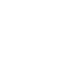### How to Work with Date Functions in Microsoft Excel 2007

Working with dates in Microsoft Excel can be a confusing task for anyone new to the system, but date calculations are so common in business and life it is bound to be something you must face at some point in your spreadsheet work. In this quick guide, we will look at how you can easily manipulate dates in Excel to give you the answers you need.

### How Excel stores Dates

In Excel a date is stored as a number which represents the number of days since 0-Jan-1900. For example the date 30-Nov-2003 will be stored as 37955 because there are 37955 days between 30-Nov-2003 and 0-Jan-1900.

This means that Excel can do all kinds of clever things, such as compare dates, add or subtract, and also give you information about the dates such as which day of the week a date lands on, and so on.

### Working with Excel Date Functions

To really explore the Microsoft Excel date functions, we will take a look at the following things:

• Find the date of the first day of the month
• Find the date of last day of the month
• Find the date of the first day of the previous month
• Find the date of the second Wednesday of the month

The first thing we have to learn before we start manipulating dates are the YEAR, MONTH and DAY functions.

### Year, Month and Day Functions

• The YEAR function returns a four digit year (a number from 1900 to 9999) for a given date value.
• The MONTH function returns the month (a number from 1 to 12) for a given date value.
• The DAY function returns the day of the month (a number from 1 to 31) for a given date value.

So, if we have the date 14-Jun-2010 in cell A1 we would see:

OK, that’s cool, let’s start the DATE manipulations.

### Working with Dates

So we have the date 14-Jun-2010 in cell A1. If we want to find the date of the first day of the month, all we have to do is create a date with the same Year and Month as A1 and change the day to 1. Simple, right?What can we do if we want to find the first day of the previous month?

We already know how to find the first day (just put 1 in the DAY argument), so for the previous month we just subtract 1 from the MONTH. The YEAR will stay the same, and there you go.Note: This works even for dates in January because Excel’s Date function can handle 0 and negative numbers correctly

To find the last day of the month is a little bit trickier. The solution is not straight forward as before because each month has a different number of days. This is where understanding how DATE is stored makes the difference.

We know that DATE is actually a number, so why not subtract 1 from the first day of the next month?

We use the same Year as A1, Add 1 to the month, and change the day to 1, this will give us the first day of the following month (1-Jul-2010), now we subtract 1 (remember, the DATE is stored as a number) and we get the last day of the previous month.OK, now we are getting fancy! Let’s find the second Wednesday of the month …

This task is a bit more complex, so we’ll take it step by step.

First we find the first day of the month:

Yahoo! It’s a Wednesday!

### Summary

We have only really touched on the full functionality that Excel can offer when it comes to dates, but I hope this article goes some way to helping you get your head around these powerful functions. Do you use dates a lot in your Excel work? Got any alternative solutions to the tasks we went through above? Please do let us know .

Make sure your read our earlier article on how to create PowerPoint charts from an Excel Data set.

### 1 comment

1.Pete Clucas says:

I have a template Excel Spread sheet I have been using to record monthly finance payments. The payments now need to be w=switched to weekly payments but I can’t work out how to change the formula for the dates,
Formula is currently set at =IF(D176,DATE((((YEAR(D176)-1900)+1900)-1900),MONTH(D176)+1,IF(DAY(D176)>DAY(DATE((((YEAR(D176)-1900)+1900)-1900),MONTH(D176)+2,1)-1),DAY(DATE((((YEAR(D176)-1900)+1900)-1900),MONTH(D176)+2,1)-1),DAY(F\$8))),””).
Any Ideas on how do I change this formula so the date will change weekly instead of monthly?